Home > A2C > Chapter 9 > Lesson 9.1.1 > Problem9-12

9-12.
1. A table can be used as a useful tool for finding some inverse functions. When the function has only one x in it, the function can be described with a sequence of operations, each applied to the previous result. Consider the following table for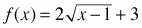. Homework Help ✎

 1st 2nd 3rd 4th What f does to x: subtracts 1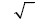multiplies by 2 adds 3

Since the inverse must undo these operations, in the opposite order, the table for f 1(x) would look like the one below.

 1st 2nd 3rd 4th What does f -1 to x: subtracts 3 divides by 2 ()2 adds 1
1. Copy and complete the following table for g1(x) if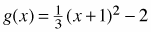1st 2nd 3rd 4th What g does to x: adds 1 ( )2 divides by 3 subtracts 2 What g−1 does to x:
2. Write the equations for f −1(x) and g1(x).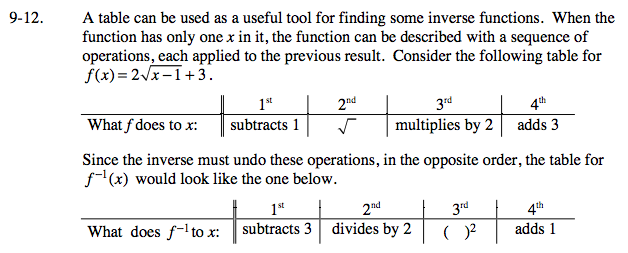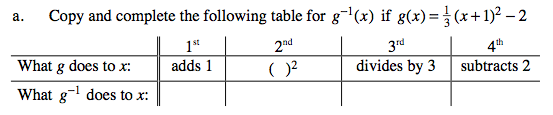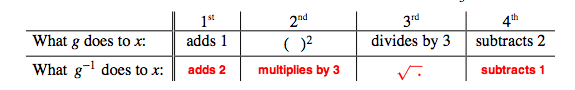Look at the table above.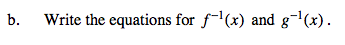$f^{-1}(x)=\left(\frac{x-3}{2}\right)^{2}+1$